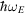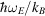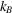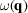International
Tables for
Crystallography
Volume B
Reciprocal space
Edited by U. Shmueli

International Tables for Crystallography (2006). Vol. B, ch. 4.1, p. 400   | 1 | 2 |

## Section 4.1.1. Introduction

B. T. M. Willisa*

aChemical Crystallography Laboratory, University of Oxford, 9 Parks Road, Oxford OX1 3PD, England
Correspondence e-mail: bertram.willis@chemcryst.ox.ac.uk

### 4.1.1. Introduction

| top | pdf |

Thermal motion of the atoms in a crystal gives rise to a reduction in the intensities of the Bragg reflections and to a diffuse distribution of non-Bragg scattering in the rest of reciprocal space. This distribution is known as thermal diffuse scattering (TDS). Measurement and analysis of TDS gives information about the lattice dynamics of the crystal, i.e. about the small oscillatory displacements of the atoms from their equilibrium positions which arise from thermal excitations. Lattice-dynamical models form the basis for interpreting many physical properties – for example, specific heat and thermal conductivity – which cannot be explained by a static model of the crystal.

Reference to a lattice-dynamical model is found in Newton's Principia, which contains a discussion of the vibrations of a linear chain of equidistant mass points connected by springs. The model was used to estimate the speed of sound in air. The vibrational properties of a one-dimensional crystal treated as a linear chain of atoms provide the starting point for several modern treatises on the lattice dynamics of crystals.

The classical theory of the dynamics of three-dimensional crystals is based on the treatment of Born & von Kármán (1912, 1913). In this theory, the restoring force on an atom is determined not by the displacement of the atom from its equilibrium position, but by its displacement relative to its neighbours. The atomic motion is then considered in terms of travelling waves, or `lattice vibrations', extending throughout the whole crystal. These waves are the normal modes of vibration, in which each mode is characterized by a wavevector q, an angular frequency ω(q) and certain polarization properties.

For twenty years after its publication the Born–von Kármán treatment was eclipsed by the theory of Debye (1912). In the Debye theory the crystal is treated as a continuous medium instead of a discrete array of atoms. The theory gives a reasonable fit to the integral vibrational properties (for example, the specific heat or the atomic temperature factor) of simple monatomic crystals. It fails to account for the form of the frequency distribution function which relates the number of modes and their frequency.

An even simpler model than Debye's is due to Einstein (1907), who considered the atoms in the crystal to be vibrating independently of each other and with the same frequency ω E. By quantizing the energy of each atom in units of, Einstein showed that the specific heat falls to zero at T = 0 K and rises asymptotically to the Dulong and Petit value for T much larger than. (is Planck's constant divided byandis Boltzmann's constant.) His theory accounts satisfactorily for the breakdown of equipartition of energy at low temperatures, but it predicts a more rapid fall-off of specific heat with decreasing temperature than is observed.

Deficiencies in the Debye theory were noted by Blackman (1937), who showed that they are overcome satisfactorily using the more rigorous Born–von Kármán theory. Extensive X-ray studies of Laval (1939) on simple structures such as sylvine, aluminium and diamond showed that the detailed features of the TDS could only be explained in terms of the Born–von Kármán theory. The X-ray work on aluminium was developed further by Olmer (1948) and by Walker (1956) to derive the phonon dispersion relations (see Section 4.1.5) along various symmetry directions in the crystal.

It is possible to measure the vibrational frequencies directly with X-rays, but such measurements are very difficult as lattice vibrational energies are many orders of magnitude less than X-ray energies. The situation is much more favourable with thermal neutrons because their wavelength is comparable with interatomic spacings and their energy is comparable with a quantum of vibrational energy (or phonon). The neutron beam is scattered inelastically by the lattice vibrations, exchanging energy with the phonons. By measuring the energy change for different directions of the scattered beam, the dispersion relationscan be determined. Brockhouse & Stewart (1958) reported the first dispersion curves to be derived in this way; since then the neutron technique has become the principal experimental method for obtaining detailed information about lattice vibrations.

In this chapter we shall describe briefly the standard treatment of the lattice dynamics of crystals. There follows a section on the theory of the scattering of X-rays by lattice vibrations, and a similar section on the scattering of thermal neutrons. We then refer briefly to experimental work with X-rays and neutrons. The final section is concerned with the measurement of elastic constants: these constants are required in calculating the TDS correction to measured Bragg intensities (see Section 7.4.2of IT C, 2004).

### References

International Tables for Crystallography (2004). Vol. C. Mathematical, physical and chemical tables, edited by E. Prince. Dordrecht: Kluwer Academic Publishers.
Blackman, M. (1937). On the vibrational spectrum of a three-dimensional lattice. Proc. R. Soc. London Ser. A, 159, 416–431.
Born, M. & von Kármán, T. (1912). Über Schwingungen in Raumgittern. Phys. Z. 13, 279–309.
Born, M. & von Kármán, T. (1913). Zur Theorie der spezifischen Wärme. Phys. Z. 14, 15–19.
Brockhouse, B. N. & Stewart, A. T. (1958). Normal modes of aluminum by neutron spectrometry. Rev. Mod. Phys. 30, 236–249.
Debye, P. (1912). Zur Theorie der spezifischen Wärme. Ann. Phys. (Leipzig), 39, 789–839.
Einstein, A. (1907). Die Plancksche Theorie der Strahlung und die Theorie der spezifisichen Wärme. Ann. Phys. (Leipzig), 22, 180–190, 800.
Laval, J. (1939). Etude éxperimentale de la diffusion des rayons X par les cristaux. Bull. Soc. Fr. Minéral. 62, 137–253.
Olmer, P. (1948). Dispersion des vitesses des ondes acoustiques dans l'aluminium. Acta Cryst. 1, 57–63.
Walker, C. B. (1956). X-ray study of lattice vibrations in aluminum. Phys. Rev. 103, 547–557.